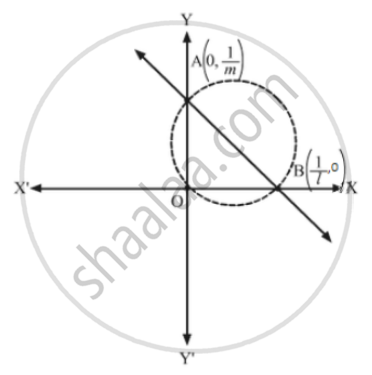Advertisement Remove all ads

# Find the Equation of the Circle Which Circumscribes the Triangle Formed by the Lines X = 0, Y = 0 and Lx + My = 1. - Mathematics

Find the equation of the circle which circumscribes the triangle formed by the lines x = 0, y = 0 and lx + my = 1.

Advertisement Remove all ads

#### SolutionThe coordinates of A and B are

$\left( 0, \frac{1}{m} \right) and \left( \frac{1}{l}, 0 \right)$
Here, the end points of the diameter of the circumcircle are A and B.
∴ Required equation of the circle:
$\left( x - 0 \right)\left( x - \frac{1}{l} \right) + \left( y - \frac{1}{m} \right)\left( y - 0 \right) = 0$
$\Rightarrow x^2 - \frac{x}{l} + y^2 - \frac{y}{m} = 0$
Concept: Circle - Standard Equation of a Circle
Is there an error in this question or solution?
Advertisement Remove all ads

#### APPEARS IN

RD Sharma Class 11 Mathematics Textbook
Chapter 24 The circle
Exercise 24.3 | Q 11 | Page 38
Advertisement Remove all ads
Advertisement Remove all ads
Share
Notifications

View all notifications

Forgot password?